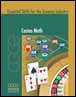# Numeracy

## Multiplying & Dividing Fractions – Concept [Video – 14:30] (2011)This math video tutorial introduces the principles for multiplying and dividing with fractions.

The instructor points out that multiplication and division of fractions, unlike addition and subtraction, do not require a common denominator.

He uses several problems to illustrate the two operations and also explains how to reduce fractions to their simplest form at various stages of the calculations.

2012-03-20

## Adding & Subtracting Fractions Part 2 – Practice [Video – 6:50] (2011)This video tutorial gives learners a chance to practise doing math problems involving fractions that don’t have the same denominator.

The tutorial includes three problems. Learners can pause the video while they work out the problems on their own, then restart it to see the instructor’s detailed solutions.

Each problem requires that the learner find a common denominator before completing the calculations.

2012-03-13

## Adding & Subtracting Fractions Part 2 – Concept [Video – 10:01] (2011)In this math video tutorial, learners deal with more complicated operations involving fractions.

The instructor takes viewers through a number of problems involving fractions that do not have the same denominator. The learner must find a common denominator before going on to the next stage of the problem

2012-03-13

## Adding & Subtracting Fractions Part 1 – Practice [Video – 3:35] (2011)This math video tutorial offers learners a chance to practise their skills at adding and subtracting fractions.

The video includes three fairly simply problems. In each example, all the fractions have the same denominator.

2012-03-07

## Adding & Subtracting Fractions Part 1 – Concept [Video – 10:46] (2011)This video provides an introduction to adding and subtracting fractions, beginning with a review of the terms numerator and denominator.

The instructor goes through the steps for solving three problems, including reducing the resulting fraction to its simplest form.

The three problems are straightforward because each of the fractions involved has the same denominator.

2012-03-07

## Order of Operations Part 2 – Practice [Video – 7:09] (2011)The order of operations specifies that the multiplication and division components of a math problem must be completed before carrying out the addition and subtraction work. It also specifies that calculations contained within brackets must be completed first.

This video tutorial reinforces that concept by providing three mathematics problems to solve. Each problem is more challenging than the one before.

2012-03-01

## Order of Operations Part 2 – Concept [Video – 10:08] (2011)This short video tutorial deals with the concept of order of operations in mathematics problems and builds on the material covered in earlier lessons.

2012-03-01

## Order of Operations Part 1 – Practice [Video – 5:31] (2011)When a mathematics problem contains some combination of addition, subtraction, multiplication or division, the order of operations specifies that the multiplication and division components must be completed first, before carrying out the addition and subtraction work.

This video tutorial reinforces that concept by providing three mathematics problems to solve. The problems get more challenging as the tutorial progresses.

2012-02-22

## Order of Operations Part 1 – Concept [Video – 6:33] (2011)This brief video tutorial explains the concept of order of operations in mathematics problems.

2012-02-22

## Casino Math: Essential Skills for the Gaming Industry (2010)This workbook is part of a series developed through a two-year project initiated by the Canadian Gaming Centre of Excellence (CGCE), a subsidiary of Manitoba Lotteries. While the project focused on meeting the training needs of Aboriginal or new Canadians, the material could be relevant for any new or potential employees in the gaming industry.